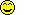<![CDATA[Math Is Fun Forum / Factorials, Hyperfactorials and Superfactorials]]> 2016-12-14T05:37:40Z FluxBB http://www.mathisfunforum.com/viewtopic.php?id=6117 <![CDATA[Re: Factorials, Hyperfactorials and Superfactorials]]> That is true since Knuth invented the arrow notation.

]]>
http://www.mathisfunforum.com/profile.php?id=33790 2016-12-14T05:37:40Z http://www.mathisfunforum.com/viewtopic.php?pid=391463#p391463
<![CDATA[Re: Factorials, Hyperfactorials and Superfactorials]]> In Knuth and Graham's book, Concrete Mathematics, the authors use notations like falling factorials for discrete mathematics.

]]>
http://www.mathisfunforum.com/profile.php?id=95904 2016-12-14T03:52:47Z http://www.mathisfunforum.com/viewtopic.php?pid=391460#p391460
<![CDATA[Re: Factorials, Hyperfactorials and Superfactorials]]> Number theory maybe.

]]>
http://www.mathisfunforum.com/profile.php?id=33790 2016-12-13T17:23:55Z http://www.mathisfunforum.com/viewtopic.php?pid=391427#p391427
<![CDATA[Re: Factorials, Hyperfactorials and Superfactorials]]> Where are these used? In Physics?

]]>
http://www.mathisfunforum.com/profile.php?id=208001 2016-12-13T16:33:27Z http://www.mathisfunforum.com/viewtopic.php?pid=391426#p391426
<![CDATA[Re: Factorials, Hyperfactorials and Superfactorials]]> Neil Sloane and Simon Plouffe defined a superfactorial in The Encyclopedia of Integer Sequences (Academic Press, 1995) to be the product of the first n factorials. So the superfactorial of 4 is
sf(4)=4!*3!*2!*1!=288 and
sf(n)=n!*(n-1)!*(n-2)!..........2!*1!

Alternative definition

Clifford Pickover in his 1995 book Keys to Infinity used a new notation, n\$, to define the superfactorial
x\$=(x!)^(x!)^(x!)^(x!).....{x! times}

Source:Wikipedia

]]>
http://www.mathisfunforum.com/profile.php?id=213230 2016-12-04T10:34:58Z http://www.mathisfunforum.com/viewtopic.php?pid=391198#p391198
<![CDATA[Re: Factorials, Hyperfactorials and Superfactorials]]> Toast,
This page gives details of Knuth's up-arrow notation. The operation becomes much more complicated when the number of up-arrows is more.

]]>
http://www.mathisfunforum.com/profile.php?id=682 2007-02-22T04:28:21Z http://www.mathisfunforum.com/viewtopic.php?pid=60133#p60133
<![CDATA[Re: Factorials, Hyperfactorials and Superfactorials]]> Zhylliolom wrote:
Toast wrote:

In Knuth's upper arrow notation, how many powers do you raise n to?

means raise a to itself n-1 times. For example,

Then basically there is a "tower" of n a's.

I can post more on this notation once I figure out how to make the LaTeX work here. (does someone know how to do underbraces in this forum? I can't see you get it to work)

]]>
http://www.mathisfunforum.com/profile.php?id=3022 2007-02-21T21:15:09Z http://www.mathisfunforum.com/viewtopic.php?pid=60116#p60116
<![CDATA[Re: Factorials, Hyperfactorials and Superfactorials]]> Toast wrote:

In Knuth's upper arrow notation, how many powers do you raise n to?

means raise a to itself n-1 times. For example,

Then basically there is a "tower" of n a's.

I can post more on this notation once I figure out how to make the LaTeX work here. (does someone know how to do underbraces in this forum? I can't see you get it to work)

]]>
http://www.mathisfunforum.com/profile.php?id=1171 2007-02-21T08:16:43Z http://www.mathisfunforum.com/viewtopic.php?pid=60048#p60048
<![CDATA[Re: Factorials, Hyperfactorials and Superfactorials]]> ganesh wrote:

Yes, you are correct, Patrick!
That is what it is.
I had even given examples of H(1)=1,
H(2)=4, H(3)=1.2².3³=1 x 4 x 27=108.

Oh well, I guess I'm turning blind.. Thanks for sharing the knowledge thoughHad never heard of hyperfactorials before!

]]>
http://www.mathisfunforum.com/profile.php?id=3022 2007-02-21T07:45:38Z http://www.mathisfunforum.com/viewtopic.php?pid=60047#p60047
<![CDATA[Re: Factorials, Hyperfactorials and Superfactorials]]> In Knuth's upper arrow notation, how many powers do you raise n to?

]]>
http://www.mathisfunforum.com/profile.php?id=5267 2007-02-21T07:12:02Z http://www.mathisfunforum.com/viewtopic.php?pid=60044#p60044
<![CDATA[Re: Factorials, Hyperfactorials and Superfactorials]]> Yes, you are correct, Patrick!
That is what it is.
I had even given examples of H(1)=1,
H(2)=4, H(3)=1.2².3³=1 x 4 x 27=108.

]]>
http://www.mathisfunforum.com/profile.php?id=682 2007-02-21T04:09:46Z http://www.mathisfunforum.com/viewtopic.php?pid=60032#p60032
<![CDATA[Re: Factorials, Hyperfactorials and Superfactorials]]> Is the hyperfactorial meant to be:
? If it isn't, then I'm not sure I understand your notation

]]>
http://www.mathisfunforum.com/profile.php?id=3022 2007-02-20T16:58:25Z http://www.mathisfunforum.com/viewtopic.php?pid=59986#p59986
<![CDATA[Factorials, Hyperfactorials and Superfactorials]]> The factorial notation must be familiar to most of you.

n! (read as n factorial) is defined as
n!=n(n-1)(n-2)(n-3)...........4 x 3 x 2 x 1.
Thus, 2!=2 x 1 = 2
3! = 3 x 2 x 1 = 6,
4! = 4 x 3 x 2 x 1 = 24
5! = 5 x 4 x 3 x 2 x 1 = 120
6! = 6 x 5 x 4 x 3 x 2 x 1 = 720 and so on.
Facotrials are useful in Combinatorics (Permutations, Combinations etc.), Probability theory, Binomial theorem, Calculus etc.

Hyperfactorial is defined as

Thus,
H(1) = 1,
H(2) = 4,
H(3) = 108 and so on.

Finally, the Superfactorial.

Clifford Pickover in his 1995 book Keys to Infinity defined the superfactorial of n as

When expressed in Knuth's up-arrow notation.

n\$=n!^^n!

For example,

The function grows very rapidly and as n increases, the tower of powers increases at a very quick rate.

100\$ would have more powers in the tower than a Googol! And
1000\$ would have more powers in the tower than

.
These are extremely large numbers, and absolutely useless to a common man!
That is because a person may never encounter a number greater than

for most of his/her life, and certainly never ever think of anything near

unless he's/she's a mathematician!]]>
http://www.mathisfunforum.com/profile.php?id=682 2007-02-20T14:37:41Z http://www.mathisfunforum.com/viewtopic.php?pid=59966#p59966